International
Tables for
Crystallography
Volume C
Mathematical, physical and chemical tables
Edited by E. Prince

International Tables for Crystallography (2006). Vol. C, ch. 9.8, pp. 943-944
https://doi.org/10.1107/97809553602060000624

## Appendix A9.8.1. Glossary of symbols

T. Janssen,a A. Janner,a A. Looijenga-Vosb and P. M. de Wolffc

aInstitute for Theoretical Physics, University of Nijmegen, Toernooiveld, NL-6525 ED Nijmegen, The Netherlands,bRoland Holstlaan 908, NL-2624 JK Delft, The Netherlands, and cMeermanstraat 126, 2614 AM, Delft, The Netherlands

 M* Vector module in m-dimensional reciprocal space (m = 1, 2, 3; normally m = 3), isomorphic to Zn with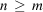. The dimension of M* is m, its rank n.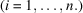Basis of a vector module M* of rank n; if n = 4 and q is modulation wavevector (the n = 4 case is restricted in what follows to modulated crystals), the basis of M* is chosen as a*, b*, c*, q, with a*, b*, c* a basis of the lattice of main reflections. Λ* Lattice of main reflections, m-dimensional reciprocal lattice. a*, b*, c* (Conventional) basis of Λ* for m = 3. Λ Direct m-dimensional lattice, dual to Λ*.Superspace; Euclidean space of dimension n = m + d;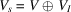. V Physical (or external) space of dimension m (m = 1,2 or 3), also indicated by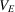.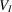Internal (or additional) space of dimension d.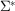Reciprocal lattice in n-dimensional space, whose orthogonal projection on V is M*.Lattice in n-dimensional superspace for which* is the reciprocal one.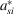Lattice basis of* in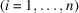; if n = 4, this basis can be chosen as {(a*, 0), (b*, 0), (c*, 0), (q, 1)} and is called standard. An equivalent notation is (q, 1) = (q, d*); for n = 3 + d, the general form of a standard basis is (a*, 0), (b*, 0), (c*, 0),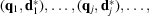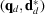.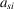(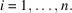) Lattice basis ofindual to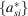; if n = 4, the standard basis is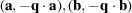,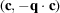, (0, 1) = (0, d); for n = 3 + d, a standard basis is dual to the standard one given above. qj Modulation wavevector(s)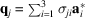; if n = 4,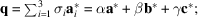σ = (α, β, γ); if n = 4,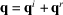, with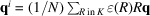, where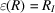, and N is the order of K. H Bragg reflections: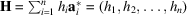; if n = 4,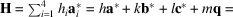(h, k, l, m).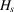Embedding of H in: for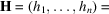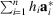, one has correspondingly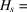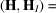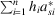.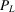Laue point group. O(m) Orthogonal group in m dimensions. R Orthogonal point-group transformation, element of O(m). K Point group, crystallographic subgroup of O(m).Superspace point-group element: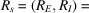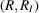element of O(m) × O(d) with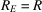external, andinternal part of, respectively; if n = 4, superspace point-group element: [R,(R)] with(R) = ±1, also written (R,).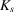Point group, crystallographic subgroup of O(m) × O(d).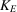External part of, crystallographic point group, subgroup of O(m) with as elements the external part transformations of.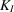Internal part of, crystallographic point group, subgroup of O(d) with as elements the internal part transformations of.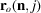Atomic positions in the basic structure: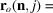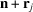with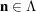. r(n, j) Atomic positions in the displacively modulated structure; (d = 1): r(n, j) =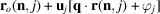. In general, however, different phases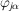may occur for different components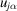along the crystallographic axes.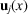Modulation function for displacive modulation with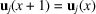.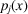Modulation function for occupation modulation with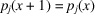. g Euclidean transformation in m dimensions; g = {R|v} element of the space group G with rotational part R and translational part v.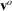Intrinsic translation part (origin independent).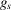Superspace group transformation (d = 1):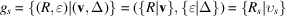element of the superspace group. In the (3 + d)-dimensional case: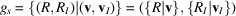.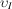Internal shift (d = 1):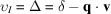. τ Intrinsic internal shift (d = 1): τ = δ −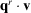.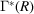Point-group transformation R with respect to a basis of M* and at the same time superspace point-group transformationwith respect to a corresponding basis of. Γ(R) Superspace point-group transformation with respect to a lattice basis ofdual to that ofleading to. The mutual relation is then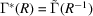with the tilde denoting transposition.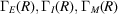external, internal, and mixed blocks of Γ(R), respectively.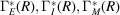external, internal, and mixed blocks of, respectively.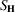Structure factor: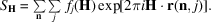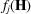Atomic scattering factor for atom j.